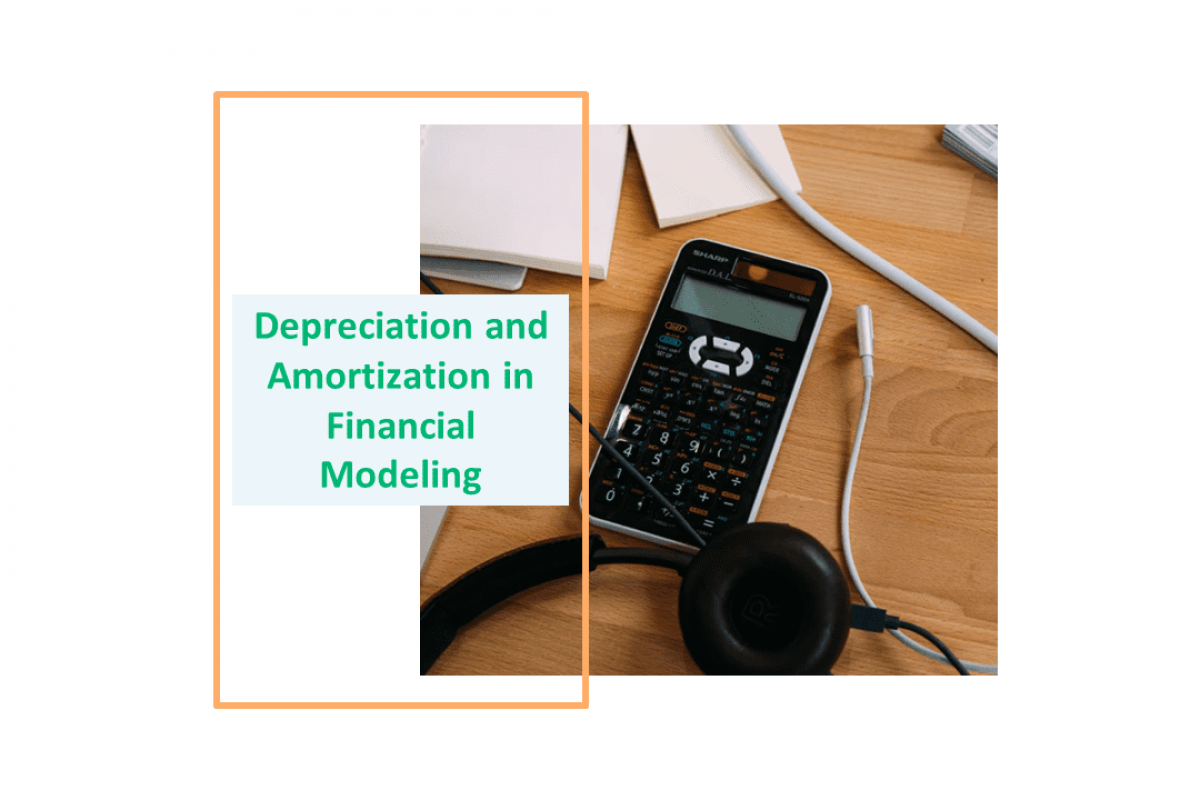# Depreciation and Amortization in Financial ModelingKeyskillset
11
July
2019### What is Depreciation and Amortization?

Depreciation refers to two aspects of the same concept. First, the actual decrease in the value of the fair value of a fixed asset. For example, a decrease in the value of equipment each year as it is used and wears. Second, the allocation in accounting statements of the original cost of the assets to periods in which the assets are used.

Fixed assets are tangible assets, meaning they are physical assets that can be touched. For instance, these are assets commonly depreciated include buildings, equipment, land, vehicles, machinery.

Amortization is an accounting technique used to periodically lower the book value of a loan or intangible asset over a set period of time. Finance professionals do that for accounting and tax purposes.

An intangible asset is an asset that lacks physical substance. For example, patents, copyright, franchises, goodwill, trademarks, and trade names.

### Amortization vs. Depreciation

We can expense the cost of business assets each year over the life of the asset. Thus, depreciation and amortization are two methods of calculating value for those business assets. We use the expense amounts as a tax deduction reducing the tax liability for the business. However, amortization refers to intangible assets whereas depreciation has to do with tangible (fixed) assets.

### Depreciation Expense and Accumulated Depreciation in Financial Statements

Depreciation expense is an income statement item. The companies account for it when they record the loss in value of their fixed assets through depreciation. Unlike other expenses, depreciation expenses are listed on income statements as a “non-cash” charge. This indicates that no money was transferred when expenses were incurred.

We record the accumulated depreciation on the balance sheet. This item reflects the total depreciation charges taken to date on a specific asset. It drops in value due to wear and tear or obsolescence.

Since the companies do not want to reduce cash on the balance sheet, depreciation expenses appear on an income statement. We add these to the accumulated depreciation account. By that, we lower the carrying value of the relevant fixed assets.

### Depreciation Calculation Methods

To calculate the depreciation we require three main inputs:

1. Useful life. This is the time period over which the organization considers the fixed asset to be productive. Beyond its useful life, the fixed asset is no longer cost-effective to continue the operation of the asset.
2. Salvage value. Post the useful life of the fixed asset, the company may consider selling it at a reduced amount. Besides, we know it as the salvage value of the asset.
3. The cost of the asset. This includes taxes, shipping, and preparation/setup expenses.

The unit of production method needs the number of units that we use during production. Let’s take a look at each type of Depreciation method in detail:

#### 1) Straight-line depreciation method

This is the simplest method of all. It involves a simple allocation of an even rate of depreciation every year over the useful life of the asset. The formula for straight-line depreciation is:

Annual Depreciation expense = (Asset cost – Residual Value) / Useful life of the asset

#### 2) Unit of Production method

This is a two-step process, unlike the straight-line method. Here, we assign equal expense rates to each unit produced. This assignment makes the method very useful in assembly for production lines. Hence, the calculation depends on the output capability of the asset rather than the number of years.

Step 1: Calculate per unit depreciation:

Per unit Depreciation = (Asset cost – Residual value) / Useful life in units of production

Step 2: Calculate the total depreciation of actual units produced: Total Depreciation Expense = Per Unit Depreciation * Units Produced

#### 3) Double declining method (accelerated depreciation)

It is one of the two common methods a company uses to account for the expenses of a fixed asset. Moreover, this is an accelerated depreciation method. As the name suggests, it counts expense twice as much as the book value of the asset every year. The formula is:

Depreciation = 2 * Straight line depreciation percent * book value at the beginning of the accounting period

Book value = Cost of the asset – accumulated depreciation

When a company uses an accelerated depreciation method, it lowers the value of its total assets on its balance sheet earlier in the life of those assets. Thus, many companies employ accelerated depreciation methods when they have assets that they expect to be more productive in their early years.

Accelerated depreciation helps companies shield income from taxes. After all, the higher the depreciation expense, the lower is the net income.  However, high depreciation expenses that we record now, mean fewer depreciation expenses recorded later. Thus, we expect a higher net income and taxes at the end of the asset‘s useful life. Essentially, this means that accelerated depreciation defers taxes for companies rather than helps companies avoid taxes.

### How to calculate the Amortization?

The formula for calculating the amortization on an intangible asset is similar to the one used for calculating straight-line depreciation. In other words, you divide the initial cost of the intangible asset by the estimated useful life of the intangible asset. Since we acquired the asset, the amount of amortization accumulated appears on the balance sheet as a deduction under the amortized asset.

The Amortization Formula: Initial Cost / Useful Life = Amortization per Year

### Conclusion

• Depreciation is how you measure the loss in value of an asset.
• However, it is an accounting figure only; it doesn’t involve a cash outlay.
• whereas, amortization is how you measure the loss in value of an intangible asset’s expense.

If you want to improve your Financial Modeling skills, try our keySkillset educational game to make a positive change in your career.